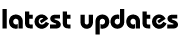Ganit Mantra Series : Circles-2 ( Tangents and Chords to the circle)

Ganit Mantra Series : Circles-2 ( Tangents and Chords to the Circle)

A line touches the circle at only one point. That line is called Tangent to the circle .We first consider the line  y= mx+ c   be a tangent to the circle,then we can solve the simultaneous system of two equations of line and circle  and put the discriminant of the resulting quadratic equation  to obtain the condition for tangency. Here, we follow an alternative approach. We use the simple geometric fact that if a line L is a tangent to a circle S, then the perpendicular distance of the centre of S from L must equal the radius of S….

TO KNOW DETAIL CLICK THE ABOVE LINK ….My Great Web page